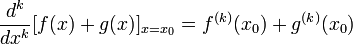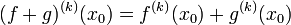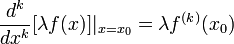# Repeated differentiation is linear

View other differentiation rules

## Statement

For$k$ a positive integer, denote by$f^{(k)}$ the function obtained by differentiating$f$ a total of$k$ times. The operation$f \mapsto f^{(k)}$ is a linear operator. We give two equivalent ways of stating this below.

### In terms of additivity and pulling out scalars

The following are true:

• Repeated differentiation is additive, or$k^{th}$ derivative of sum is sum of derivatives: If$f$ and$g$ are functions that are both differentiable at$x = x_0$, we have:$\frac{d^k}{dx^k}[f(x) + g(x)]_{x = x_0} = f^{(k)}(x_0) + g^{(k)}(x_0)$

or equivalently:$(f + g)^{(k)}(x_0) = f^{(k)}(x_0) + g^{(k)}(x_0)$

In point-free notation:$(f + g)^{(k)} = f^{(k)} + g^{(k)}$

• Constants (also called scalars) can be pulled out of differentiations: If$f$ is differentiable at$x = x_0$ and$\lambda$ is a real number, then:$\frac{d^k}{dx^k}[\lambda f(x)]|_{x = x_0} = \lambda f^{(k)}(x_0)$

### In terms of generalized linearity

Suppose$f_1, f_2, \dots, f_n$ are functions that are all differentiable at a point$x_0$ and$a_1, a_2, \dots, a_n$ are real numbers. Then:$\frac{d^k}{dx^k}[a_1f_1(x) + a_2f_2(x) + \dots + a_nf_n(x)]|_{x = x_0} = a_1f_1^{(k)}(x_0) + a_2f_2^{(k)}(x_0) + \dots + a_nf_n^{(k)}(x_0)$